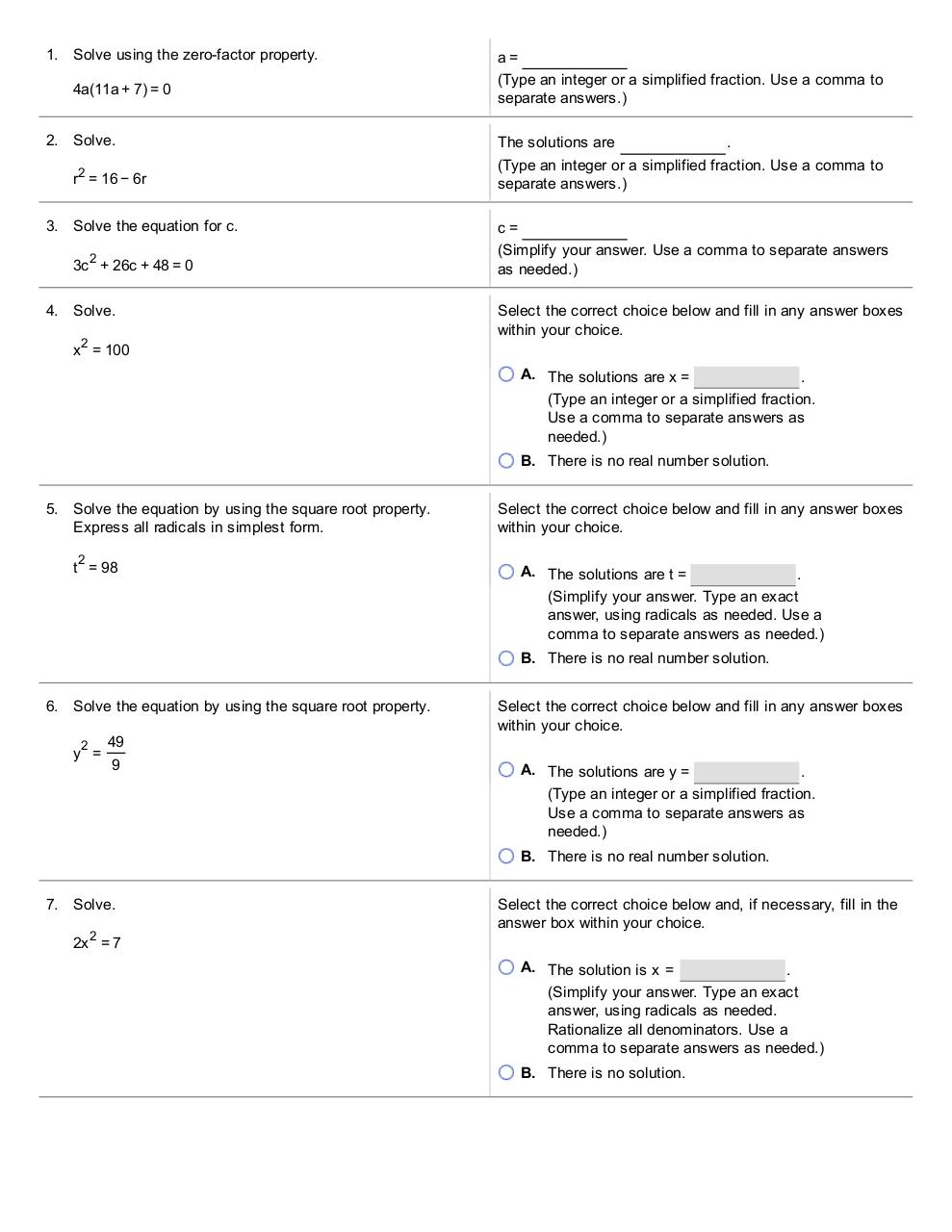# College Level.pdfPage 1 2 3 4 5 6 7 8 9 10

#### Text preview

1. Solve using the zero­factor property.
4a(11a + 7 ) = 0
2. Solve.
r2 = 16 − 6r
3. Solve the equation for c .
2

3c + 26c + 48 = 0
4. Solve.
x 2 = 100

a=
(Type an integer or a simplified fraction. Use a comma to
The solutions are
.
(Type an integer or a simplified fraction. Use a comma to
c=
as needed.)
Select the correct choice below and fill in any answer boxes
within your choice.
A. The solutions are x =
.
(Type an integer or a simplified fraction.
Use a comma to separate answers as
needed.)
B. There is no real number solution.

5. Solve the equation by using the square root property.
Express all radicals in simplest form.
2

t = 98

Select the correct choice below and fill in any answer boxes
within your choice.
A. The solutions are t =
.
(Simplify your answer. Type an exact
comma to separate answers as needed.)
B. There is no real number solution.

6. Solve the equation by using the square root property.
2

y =

49
9

Select the correct choice below and fill in any answer boxes
within your choice.
A. The solutions are y =
.
(Type an integer or a simplified fraction.
Use a comma to separate answers as
needed.)
B. There is no real number solution.

7. Solve.
2x 2 = 7

Select the correct choice below and, if necessary, fill in the